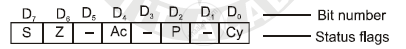# Explain Any Four Flags of 8085, Giving Example - Computer Science 2

Explain any four flags of 8085, giving example

#### Solution

8085 has five flags. Sign flag, zero flag, Auxiliary carry flag, Parity flag and Carry flag. A 8-bit register is used to represent five flags as shown in following figure.Where S - Sign flag, Z- Zero flag, Ac- Auxiliary carry flag,

P - Parity flag, Cy- Carry flag.

(1) Sign flag (S) :
After the execution of arithmetic and logic operation, if the most signification of the result is 1, then the flag is set to 1 otherwise 0. This flag is used with signed number. If MSB is 1, the number will be negative and if it is 0, the number will be positive.

(2) Zero flag(Z) :
After performing an arithmetic or logic operations, if the result is zero, then zero flag is set to 1, else it is reset. This flag is modified by the results in accumulator as well as in other registers.

(3) Auxiliary carry flag(Ac) :
In an arithmetic operation, when carry is generated from bit D3 to D4, the auxiliary carry flag is set to 1. This flag is only available internally and used for B.C.D. operations and not available for programmer.

(4) Parity flag (P) :
Parity flag is set to 1, if the result stored in accumulator contains even parity, i.e., even number of 1’s. If accumulator contains odd number of 1’s, the flag is 0.

Concept: Introduction to Microprocessors and Organization of 8085
Is there an error in this question or solution?
2014-2015 (March)

Share